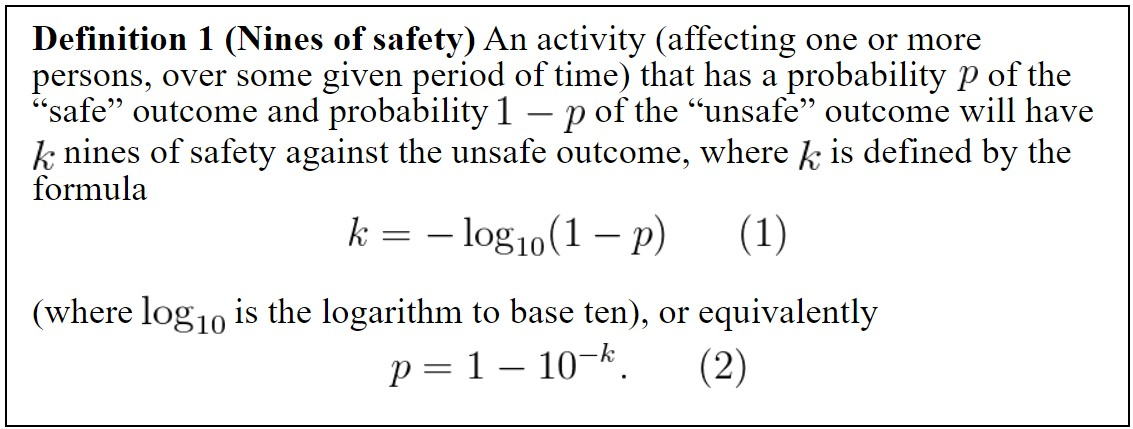# Nines of safety: a proposed unit of measurement of risk

Terence Tao in his own blog:Because of all the very different ways in which percentages could be used, I think it may make sense to propose an alternate system of units to measure one class of probabilities, namely the probabilities of avoiding some highly undesirable outcome, such as death, accident or illness. The units I propose are that of “nines“, which are already commonly used to measure availability of some service or purity of a material, but can be equally used to measure the safety (i.e., lack of risk) of some activity. Informally, nines measure how many consecutive appearances of the digit${9}$ are in the probability of successfully avoiding the negative outcome, thus

•${90\%}$ success = one nine of safety
•${99\%}$ success = two nines of safety
•${99.9\%}$ success = three nines of safety

and so forth. Using the mathematical device of logarithms, one can also assign a fractional number of nines of safety to a general probability…

More here.